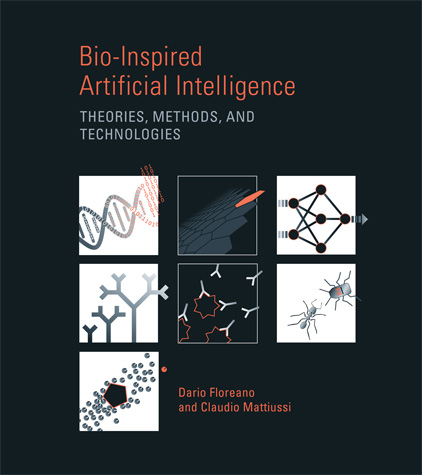MIT Press 659 pp., 290 illus. September 2008 ISBN-10: 0-262-06271-2 ISBN-13: 978-0-262-06271-8

Errata

Last update May 3, 2010
Please send any feedback, comments, and corrections to Claudio Mattiussi

Errors and clarifications

 Ch. Page Description - - 1 - - 2 - - 3 - - 4 - - 5 - - 6 - - 7 - - - -

Typos

 Ch. Page Description - - 1 - - 2 - - 3 243 Missing bracket in source citation. At the end of the caption for figure 3.46, add opening round bracket before "From Dürr et al. 2006)." [Thanks to Peter Dürr] 4 269 274 281 Typo in text. Line 7 from top: se huge –› such huge Typo in text. The axiom of the L-system whose development is represented in Figure 4.1 is d_l, not d_r. [Thanks to Ernesto Costa, Departamento de Engenharia Informática Universidade de Coimbra] Typo in text. Line 13 from bottom: remove bracket "... in figure 4.8a)" 5 - - 6 442 480 Typo in figure caption. In caption of figure 6.27: remove text "Figures 16 and 15 from AutoRobo." Missing citation (Floreano and Mondada, 1996a), 3rd line after "These questions were explored..." Also, the reference entry should be added to the bibliography: Floreano, D. and Mondada, F. (1996) Evolution of Homing Navigation in a Real Mobile Robot. IEEE Transactions on Systems, Man and Cybernetics Part B: Cybernetics, 26(3): 396-407. 7 525 Typo in formula. In section 7.2 (p. 525) the formula for a particle's velocity is specified as $v_{i}^{t+1}=av_{i}^{t}+b(x_{i}^{p}-x_{i}^{t})+c(x_{j}^{t}-x_{i}^{t})$. However, in text the sub-term that refers to the best observed position $(x_{i}^{p}-x_{i}^{t})$ is denoted as $(x_{i}^{b}-x_{i}^{t})$. In the last line on p. 525 the value $x_{i}^{p}$ is also refered to as $x_{i}^{b}$. [Thanks to Dietmar Schreiner, Institute of Computer Languages, Vienna University of Technology] 527 Typo in citation. "Landon" should be "Langdon" here and in bibliography (page 622) "Landon, W. B. and Poli, R. (2007). Evolving problems to learn about particle swarm optimizers and other search algorithms. IEEE Transactions on Evolutionary Computation, 11:561–578." 529 Typo in formula. In Section 7.3, in the formula for pheromone evaporation, add a plus operator (+) between the evaporated amount of pheromones and the newly added ones, i.e., read $\tau_{ij}^{t+1}=(1-\rho)\tau_{ij}^{t}+\Delta T_{ij}$ instead of $\tau_{ij}^{t+1}=(1-\rho)\tau_{ij}^{t}~\Delta T_{ij}$ [Thanks to Dietmar Schreiner, Institute of Computer Languages, Vienna University of Technology] 565 Missing brackets in citation: "Floreano and Nolﬁ 1997b and Floreano et al. 2001" should be "Floreano and Nolﬁ (1997b) and Floreano et al. (2001)" 569 Typo in citation. Year should be 1999 (not 1998) in citation "Nolfi and Floreano (1998)" here and in bibliography (page 631) "Nolfi, S. and Floreano, D. (1998). Co-evolving predator and prey robots: Do 'arms races' arise in artificial evolution? Artificial Life, 4:311–335."created by claudio mattiussi, 2008